## Condition for Reciprocal Two Port Network:

If the ratio of voltage at one port to the current at other port is same to the ratio if the positions of voltage and current are interchanged, then the network is said to be Condition for Reciprocal Two Port Network. The transfer impedances can be measured as shown in Fig. 6.7 (a) and (b).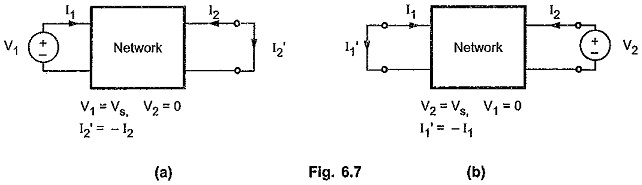#### Condition of Reciprocity for z-Parameters:

The basic equations for z-parameters are as follows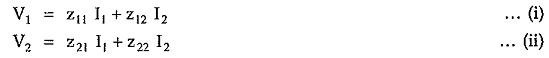Consider Fig. 6.7 (a)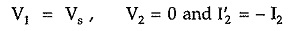Hence equation (i) can be written as,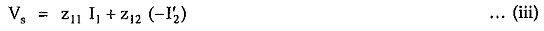Equation (ii) can be written as,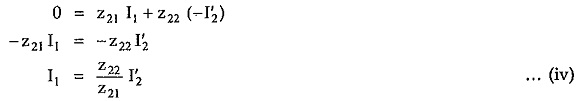Substituting value of I1 in equation (iii), we get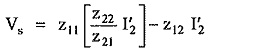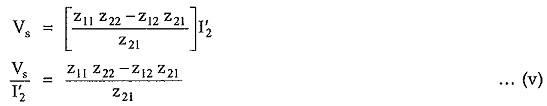Consider the Fig. 6.7 (b).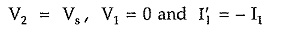Equation (i) can be written as,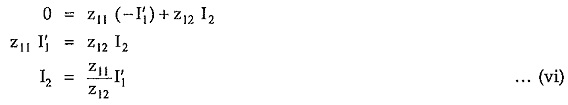Equation (ii) can be written as,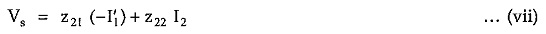Substituting value of I2 is equation (vii), we have,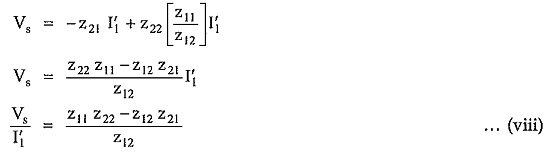For condition of reciprocity,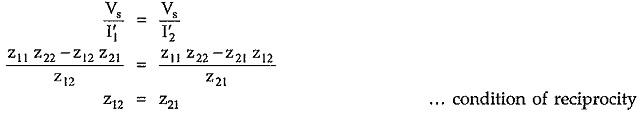#### Condition of Reciprocity for y-Parameters:

The basic equations of y-parameters are as follows,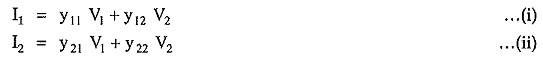Consider Fig. 6.7 (a)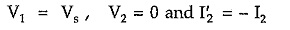Equation (i) can be written as,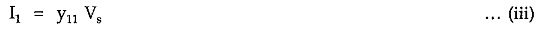Equation (ii) can be written as,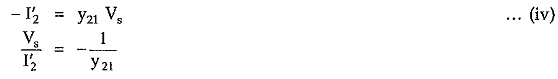Consider Fig. 6.7 (b)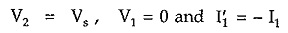Equation (i) can be written as,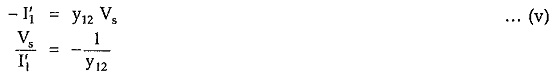The network is said to be reciprocal if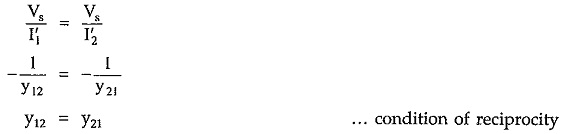#### Condition of Reciprocity for h-Parameters:

The basic equations of h-parameters are as follows,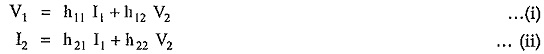Consider Fig. 6.7 (a)Equation (i) can be written as,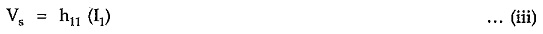Equation (ii) can be written as,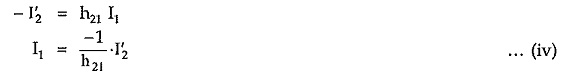Substituting value of I1 in equation (iii)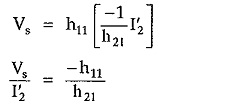Consider Fig. 6.7 (b)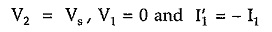Equation (i) can be written as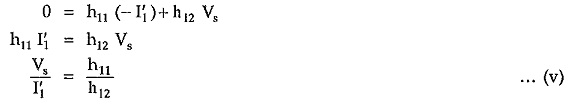The network is said to be reciprocal if,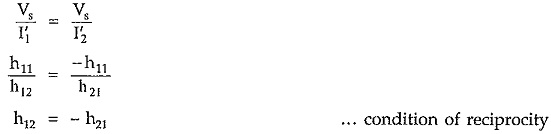#### Condition of Reciprocity for ABCD-Parameters:

The basic equations for ABCD parameters are as follows,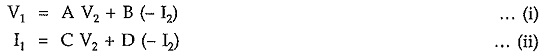Consider Fig. 6.7 (a)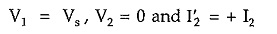Equation (i) can be written as,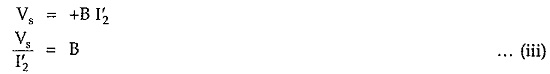Consider Fig. 6.7 (b),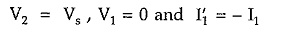Equation (i) can be written as,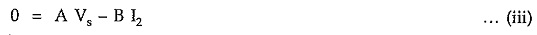Equation (ii) can be written as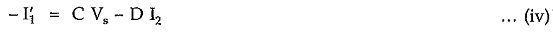From equation (iii) we have,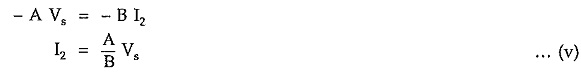Substituting this value in equation (iv) we have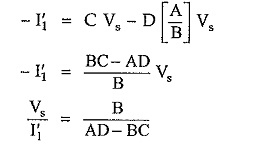The network is said to be reciprocal if,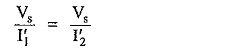Scroll to Top